# Recipes using cf¶

Version 3.15.4 for version 1.10 of the CF conventions.

Click on the keywords below to filter the recipes according to their function: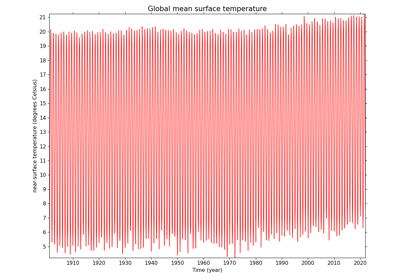Calculating global mean temperature timeseries

Calculating global mean temperature timeseries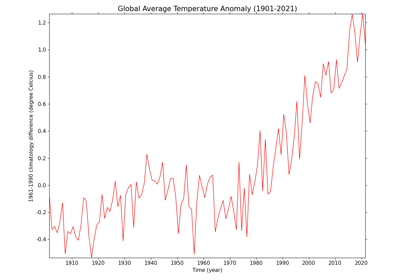Calculating and plotting the global average temperature anomalies

Calculating and plotting the global average temperature anomalies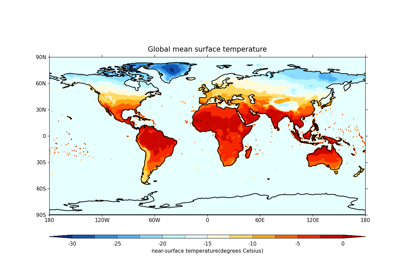Plotting global mean temperatures spatially

Plotting global mean temperatures spatially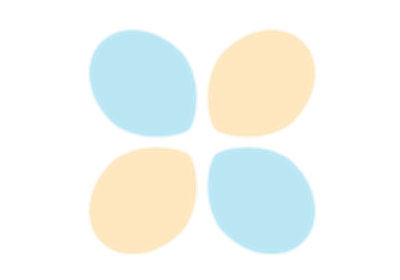Comparing two datasets with different resolutions using regridding

Comparing two datasets with different resolutions using regridding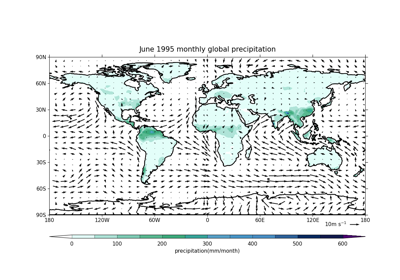Plotting wind vectors overlaid on precipitation data

Plotting wind vectors overlaid on precipitation data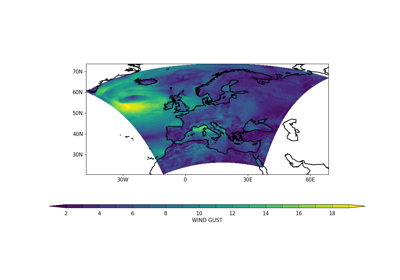Converting from rotated latitude-longitude to regular latitude-longitude

Converting from rotated latitude-longitude to regular latitude-longitude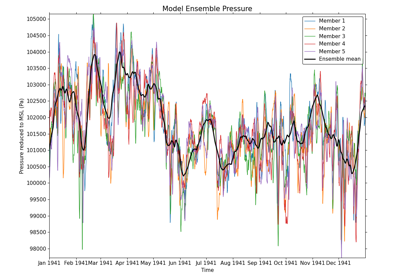Plotting members of a model ensemble

Plotting members of a model ensemble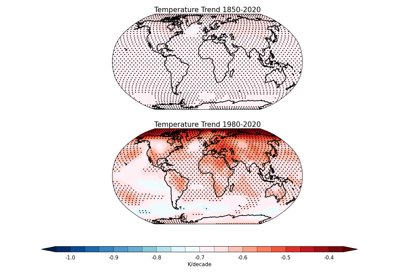Plotting statistically significant temperature trends with stippling

Plotting statistically significant temperature trends with stipplingPlotting a joint histogram

Plotting a joint histogram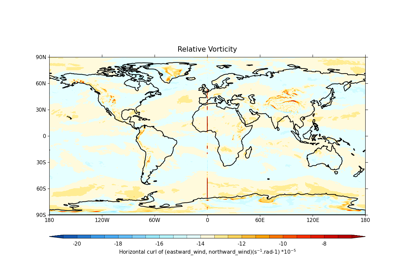Calculating and plotting the relative vorticity

Calculating and plotting the relative vorticity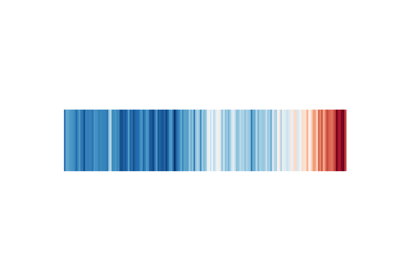Plotting the Warming Stripes

Plotting the Warming Stripes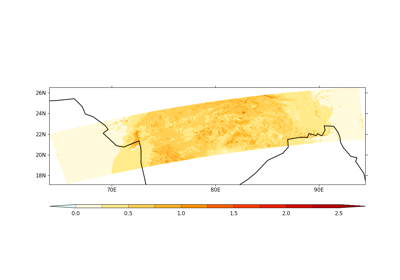Using mask to plot Aerosol Optical Depth

Using mask to plot Aerosol Optical DepthCalculate and plot the Niño 3.4 Index

Calculate and plot the Niño 3.4 Index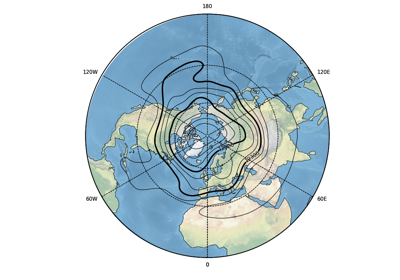Overlay Geopotential height contours over Temperature anomalies

Overlay Geopotential height contours over Temperature anomalies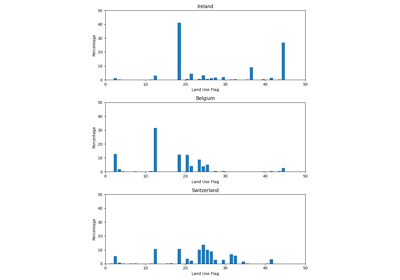Resampling Land Use Flags to a Coarser Grid

Resampling Land Use Flags to a Coarser Grid

Gallery generated by Sphinx-Gallery